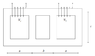# Solving Mutual Induction Exercise | Let's Get Started!

• charrotrust
In summary, the magnetic coupling between the coils is calculable using the concepts of inductance and mutual inductance. The position of the dots needed to identify the sign of the mutual inductance can be determined. If two currents flow in the two windings, the overall flow is calculated.f

#### charrotrust

Homework Statement
Mutual Induction
Relevant Equations
L=Φ/I
hello PF. I have this exercise to do but I don't know how to do it.
Let's assume that the core in the figure is made up of ferromagnetic permeability material
μ = 2000 μ0. Let a = 4 cm, b = 2 cm, c = 3 cm the geometric dimensions, S = 8 cm2 the section
transversal, N1 = 100, N2 = 300 the number of turns of the two windings.
• Calculate the inductances of the two windings and the mutual inductances between
same;
• the position of the dots needed to identify the sign of the mutual is established
inductance;
• if two currents I1 = 3 A, I2 = 4 A flow in the two windings, both entering
on the side of the dots, calculate the overall flow concatenated with the two
windings.

#### Attachments

•jhkghjkjghkjhgjlkg.PNG
3.2 KB · Views: 81
Homework Statement:: Mutual Induction
Relevant Equations:: $$$L=\frac{\phi}{Ic}$[tex] hello PF. I have this exercise to do but I don't know how to do it. Let's assume that the core in the figure is made up of ferromagnetic permeability material μ = 2000 μ0. Let a = 4 cm, b = 2 cm, c = 3 cm the geometric dimensions, S = 8 cm2 the section transversal, N1 = 100, N2 = 300 the number of turns of the two windings. • Calculate the inductances of the two windings and the mutual inductances between same; • the position of the dots needed to identify the sign of the mutual is established inductance; • if two currents I1 = 3 A, I2 = 4 A flow in the two windings, both entering on the side of the dots, calculate the overall flow concatenated with the two windings. Welcome to PhysicsForums.Per the PF rules, you need to show your best efforts to start working the problem before we can offer tutorial help. What concepts and equations should you use to calculate the magnetic coupling between the coils? Also, see the LaTeX Guide below the Edit window. The notation you are using apparently is not supported here. For the calculation of the inductance I thought I would apply the following formula which derives from the definition: [tex]L=\frac{\mu \mu _{0}N^{2}S}{l}$$
For the calculation of mutual inductions I know that:
$$\phi =M\cdot i$$
But how can I find the current?
As for the pellets, he has no idea. Do you have any ideas for me?